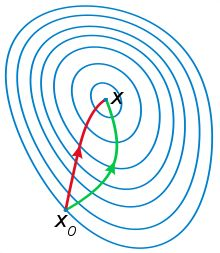关于梯度下降法、牛顿法、高斯-牛顿、LM方法的总结1.梯度下降与牛顿法

$\mathbf{b}=\mathbf{a}-\gamma \mathrm{\nabla }F\left(\mathbf{a}\right)$$\mathbf{b} = \mathbf{a}-\gamma\nabla F(\mathbf{a})$ ,$\gamma$$\gamma$是步长。

$f‘\left(x0\right)+\left(x－x0\right){f}^{″}\left(x0\right)=0$$f ‘(x0)+(x－x0)f '’(x0) = 0$

$S=\sum _{i=1}^{m}{r}_{i}^{2}$$S = \sum_{i=1}^m r_i^2$

${g}_{j}=2\sum _{i=1}^{m}{r}_{i}\frac{\mathrm{\partial }{r}_{i}}{\mathrm{\partial }{\beta }_{j}}$$g_j=2\sum_{i=1}^m r_i\frac{\partial r_i}{\partial \beta_j}$ （1）

Hessian 矩阵的元素则直接在梯度向量的基础上求导：
${H}_{jk}=2\sum _{i=1}^{m}\left(\frac{\mathrm{\partial }{r}_{i}}{\mathrm{\partial }{\beta }_{j}}\frac{\mathrm{\partial }{r}_{i}}{\mathrm{\partial }{\beta }_{k}}+{r}_{i}\frac{{\mathrm{\partial }}^{2}{r}_{i}}{\mathrm{\partial }{\beta }_{j}\mathrm{\partial }{\beta }_{k}}\right).$$H_{jk}=2\sum_{i=1}^m \left(\frac{\partial r_i}{\partial \beta_j}\frac{\partial r_i}{\partial \beta_k}+r_i\frac{\partial^2 r_i}{\partial \beta_j \partial \beta_k} \right).$

${H}_{jk}\approx 2\sum _{i=1}^{m}{J}_{ij}{J}_{ik}$$H_{jk}\approx 2\sum_{i=1}^m J_{ij}J_{ik}$ （2）

$\mathbf{g}=2{{\mathbf{J}}_{\mathbf{r}}}^{\mathrm{\top }}\mathbf{r},\phantom{\rule{1em}{0ex}}\mathbf{H}\approx 2{{\mathbf{J}}_{\mathbf{r}}}^{\mathrm{\top }}{\mathbf{J}}_{\mathbf{r}}.$$\mathbf g=2\mathbf{J_r}^\top \mathbf{r}, \quad \mathbf{H} \approx 2 \mathbf{J_r}^\top \mathbf{J_r}.$

Levenberg-Marquardt方法：

1.调整下降步伐：$\boldsymbol \beta^{s+1} = \boldsymbol \beta^s+\alpha\ \Delta. 0<\alpha<1$
2.调整下降方向：$\left({\mathbf{J}}^{\mathbf{T}}\mathbf{J}\mathbf{+}\lambda \mathbf{D}\right)\mathrm{\Delta }={\mathbf{J}}^{T}\mathbf{r}$$\left(\mathbf{J^TJ+\lambda D}\right)\Delta=\mathbf{J}^T \mathbf{r}$

$\lambda \to +\mathrm{\infty }$$\lambda\to+\infty$时：$\mathrm{\Delta }/\lambda \to {\mathbf{J}}^{T}\mathbf{r}$$\Delta/\lambda\to \mathbf{J}^T \mathbf{r}$，即方向和梯度方向一样，变成了梯度下降法。

Levenberg-Marquardt方法的好处在于可以调节:

【reference】:
http://fourier.eng.hmc.edu/e176/lectures/NM/node36.html 【理论推导很完善】
.http://blog.csdn.net/dsbatigol/article/details/12448627